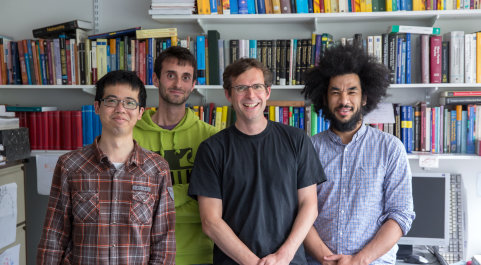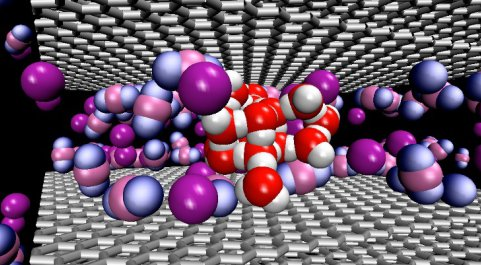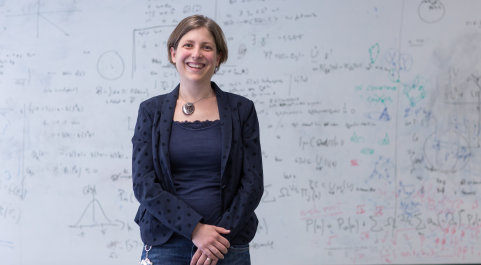# Mathematical Physics

The Mathematical Physics group is part of the Applied Mathematics and Mathematical Physics Section and is interested in the theory of condensed matter, statistical physics, complexity, biophysics, theoretical quantum dynamics and random matrices. Research interests include:

• strongly correlated electron systems
• non-equilibrium statistical mechanics including mathematical modelling of biological evolution and ecology
• protein dynamics and pattern formation
• high temperature superconductivity
• phase transitions and inhomogeneous fluids
• spintronics
• quantum fluids
• random matrix models
• quantum dynamics of ultracold atoms in optical lattices
• non-Hermitian and PT-symmetric quantum theories
• quantum-classical correspondence

Research in condensed matter theory is carried out in both the mathematics and physics departments at Imperial. There are ongoing collaborations with the Condensed Matter Theory Group in physics and the AMMP Biomathematics Group.

## Mathematical Physics